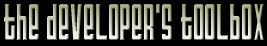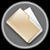This section of the archives stores flipcode's complete Developer Toolbox collection, featuring a variety of mini-articles and source code contributions from our readers.C++ Components And Sub-Components   Submitted byIt is highly important to have your code components, whether they're coded in C or in C++, to be divided into sub-components, so that if you'd like to modify something small in your code you would just change it in the sub-component instead of going through your whole source code and changing those bits, which also may cause bugs and errors. You better keep your code as generic as possible in order to be able to enjoy code reusability which means using methods to acess the members of your class. Learning about design patterns would help a great deal.

In order to show you how important it is to keep your code abstract I'll demonstrate it with a simple example. Let's say that you are about to work with triangles only. A basic triangle class would usually have three indices(each one points to it's vertex number in vertex_list).

 ```class triangle { public: int a, b, c; // the indices triangle() {} triangle(int A, int B, int C) { // constructor set(A, B, C); } void set(int A, int B, int C) { // functions to set the indices a=A; b=B; c=C; }}; ```

Simple enough? If you want to access the first vertex forming this triangle you'd just do, assuming my_triangle is variable of triangle class, v1 = vertex_list[my_triangle.a];

Now you probably got a cool model loading working very nicely and you're damn pleased with yourself. Imagine yourself after sometime finding out that rendering n-gons can be quite fast and many popular games render n-gons instead of your simple triangles. You'd find yourself in a dilemma if you're using a class like the first triangle class.

Here is how you could design your triangle class to be more general:

 ```#define MAX_VERTICES_IN_POLY 3 class triangle { int indices[MAX_VERTICES_IN_POLY]; public: triangle() {} int& set(int num) { // an accessor method return (int)get(num); // uses a sub-component to get a reference to an index of a vertex } const int& get(int num) { // don't forget to include assert.h assert(num=0 && num

We have changed the a,b,c to indices, there are already three good things with this approach:

• 1).OpenGL and Direct3D API's use an array of indices to draw a polygon.
• 2).If you want to change it to quads you would just need to change from 3 to 4 in the MAX_VERTICES_IN_POLY definition.
• 3).If you desire to have n-gons, all you'd need to do is just to make the indices a pointer (int *indices) , add another member (int nverts) and last but not least to change from num<MAX_VERTICES_IN_POLY to num<nverts.

• See how easy it is to create a generic code. If you want to get the fifth vertex of the n-gon you'd just do: v5 = vertex_list[my_triangle.get(5)];

To set a new index you'd do my_triangle.set(4) = 7; or whatsoever..

Our final class:

 ```class polygon { int *indices; int nverts; public: // constructor polygon() { indices=NULL; nverts=0; } int& set(int num) { // an accessor method return (int)get(num); // uses a sub-component to get a reference to an index of a vertex } const int& get(int num) { assert(num=0 && num

The idea is clear, some would use indices, some would store the vertices directly in triangle class, some use linked lists for storing vertices in their polygon class.The point is that using such an abstract class you could change from one to other without any problems. Try now to imagine all the pain you'd be going through if you had used the first triangle class.

Useful resources:
• www.hugi.de or www.foxfiber.net/hugi - tutorial about design patterns can be find in issue 18 and tips and tricks about c++ in issue 19.

• http://www.bruceeckel.com/ - I heard that thinking in C++ by Bruce Eckel is quite good, haven't read it though.

• Design Patterns : Elements of Reusable Object-Oriented Software
Authors: Gamma, Helm, Johnson, and Vlissides